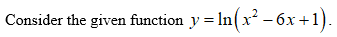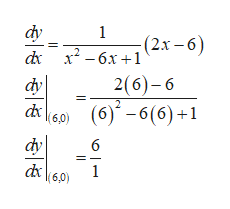Find an equation of the tangent line to the curve at the given point.y = ln(x2 − 6x + 1),    (6, 0)

Question

Find an equation of the tangent line to the curve at the given point.

y = ln(x2 − 6x + 1),    (6, 0)
Step 1Equation of the line to the curve at the given point is shown below.

Step 2

Differentiate the given function with respect to x and the slope at the given point (6, 0) is calculated as follows.help_outlineImage Transcriptioncloseфу 1 d x2-6x+1 (2.r-6) dy - бх +1 2(6)-6 2 (6)-6(6)+1 (6,0) фу d6,0 6 1 fullscreen
Step 3

Thus, the equation of the line ...

Want to see the full answer?

See Solution

Want to see this answer and more?

Our solutions are written by experts, many with advanced degrees, and available 24/7

See Solution
Tagged in

Other